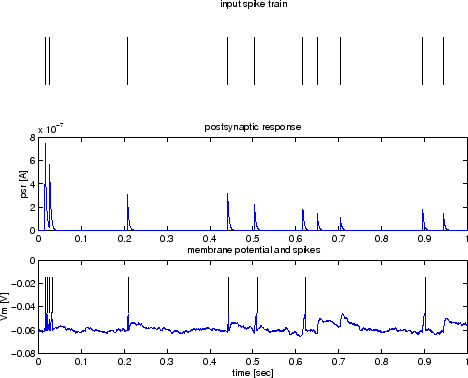Plotting the recorded traces

## Plotting the recorded traces

The traces recorded by the recorder can be obtained by the command
```
» t=csim('get',r,'traces')
```
which yields the output
```t =
channel: [1x3 struct]
```
A closer look at e.g. the first channel reveals that t.channel is a struct array with a similar structure as we have seen above for the input signal:
```>> t.channel(1)

ans =

idx: 1
fieldName: 'psr'
data: [1x2000 double]
spiking: 0
dt: 5.0000e-04
```

The output indicates that t.channel(1).data holds the psr trace (t.channel(1).fieldName) with a resolution of 0.5ms (t.channel(1).dt). See the Recorder class documentation for details abput the structure of the trace output. Similarly t.channel(2) holds the the Vm trace and t.channel(3) holds the output spike times. Hence the following command will plot the psr trace

```
» plot(t.channel(1).dt:t.channel(1).dt:Tsim,t.channel(1).data);
```
and the command
```
» stem(t.channel(3).data,ones(size(t.channel(3).data)));
```
will draw the output spike train.

Using another set of plot commands one can easily create the following figure which shows the input, the postsynaptic response and the output of the neuron (the voltage and the spikes)The full code of this example is contained as the file first_model.m in the demo directory of the CSIM package.